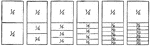### Fraction Comparisons

Rectangles comparing fraction values to one half: 1/4, 1/6, 1/8, 1/10, and 1/12.### Fraction Pie Divided into Twelfths

A circle divided into twelfths.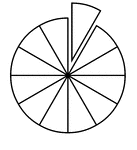### Fraction Pie Divided into Twelfths

A circle divided into twelfths with one twelfth separated.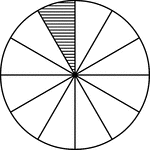### Fraction Pie Divided into Twelfths

A circle divided into twelfths with one twelfth shaded.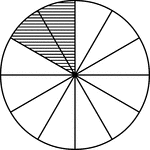### Fraction Pie Divided into Twelfths

A circle divided into twelfths with two twelfths shaded.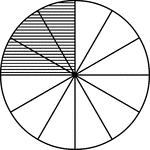### Fraction Pie Divided into Twelfths

A circle divided into twelfths with three twelfths shaded.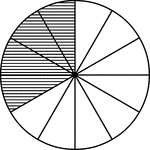### Fraction Pie Divided into Twelfths

A circle divided into twelfths with four twelfths shaded.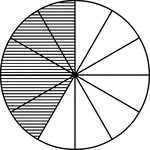### Fraction Pie Divided into Twelfths

A circle divided into twelfths with five twelfths shaded.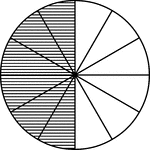### Fraction Pie Divided into Twelfths

A circle divided into twelfths with six twelfths shaded.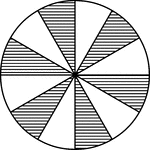### Fraction Pie Divided into Twelfths

A circle divided into twelfths with six twelfths shaded.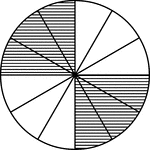### Fraction Pie Divided into Twelfths

A circle divided into twelfths with six twelfths shaded.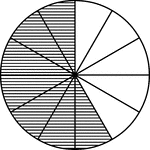### Fraction Pie Divided into Twelfths

A circle divided into twelfths with seven twelfths shaded.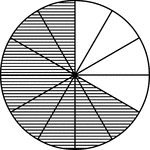### Fraction Pie Divided into Twelfths

A circle divided into twelfths with eight twelfths shaded.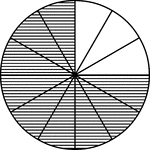### Fraction Pie Divided into Twelfths

A circle divided into twelfths with nine twelfths shaded.### Fraction Pie Divided into Twelfths

A circle divided into twelfths with ten twelfths shaded.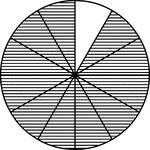### Fraction Pie Divided into Twelfths

A circle divided into twelfths with eleven twelfths shaded.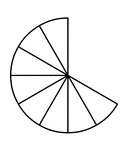### Eight Twelfths of a Fraction Pie

Eight twelfths of a circle.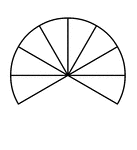### Eight Twelfths of a Fraction Pie

Eight twelfths of a circle.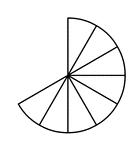### Eight Twelfths of a Fraction Pie

Eight twelfths of a circle.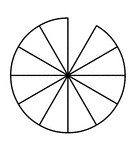### Eleven Twelfths of a Fraction Pie

Eleven twelfths of a circle.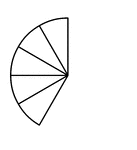### Five Twelfths of a Fraction Pie

Five twelfths of a circle.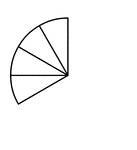### Four Twelfths of a Fraction Pie

Four twelfths of a circle.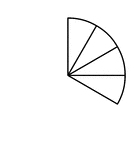### Four Twelfths of a Fraction Pie

Four twelfths of a circle.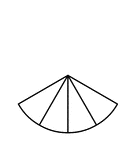### Four Twelfths of a Fraction Pie

Four twelfths of a circle.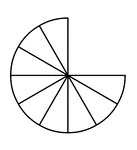### Nine Twelfths of a Fraction Pie

Nine twelfths of a circle.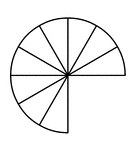### Nine Twelfths of a Fraction Pie

Nine twelfths of a circle.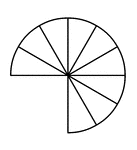### Nine Twelfths of a Fraction Pie

Nine twelfths of a circle.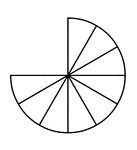### Nine Twelfths of a Fraction Pie

Nine twelfths of a circle.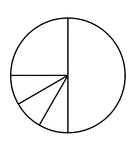### One Quarter, Three Twelfths, and One Half of a Pie Fraction

A circle subdivided into one quarter, three twelfths, and one half.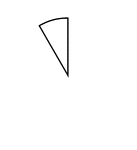### One Twelfth of a Fraction Pie

One twelfth of a circle.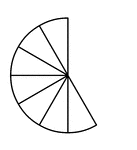### Seven Twelfths of a Fraction Pie

Seven twelfths of a circle.### Six Twelfths of a Fraction Pie

Six twelfths of a circle.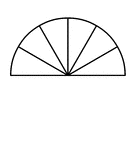### Six Twelfths of a Fraction Pie

Six twelfths of a circle.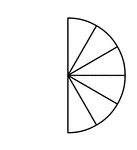### Six Twelfths of a Fraction Pie

Six twelfths of a circle.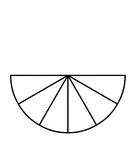### Six Twelfths of a Fraction Pie

Six twelfths of a circle.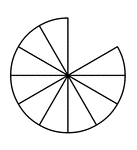### Ten Twelfths of a Fraction Pie

Ten twelfths of a circle.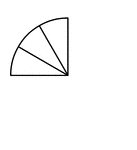### Three Twelfths of a Fraction Pie

Three twelfths of a circle.### Three Twelfths of a Fraction Pie

Three twelfths of a circle.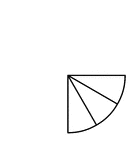### Three Twelfths of a Fraction Pie

Three twelfths of a circle.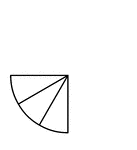### Three Twelfths of a Fraction Pie

Three twelfths of a circle.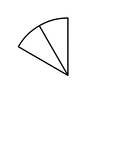### Two Twelfths of a Fraction Pie

Two twelfths of a circle.